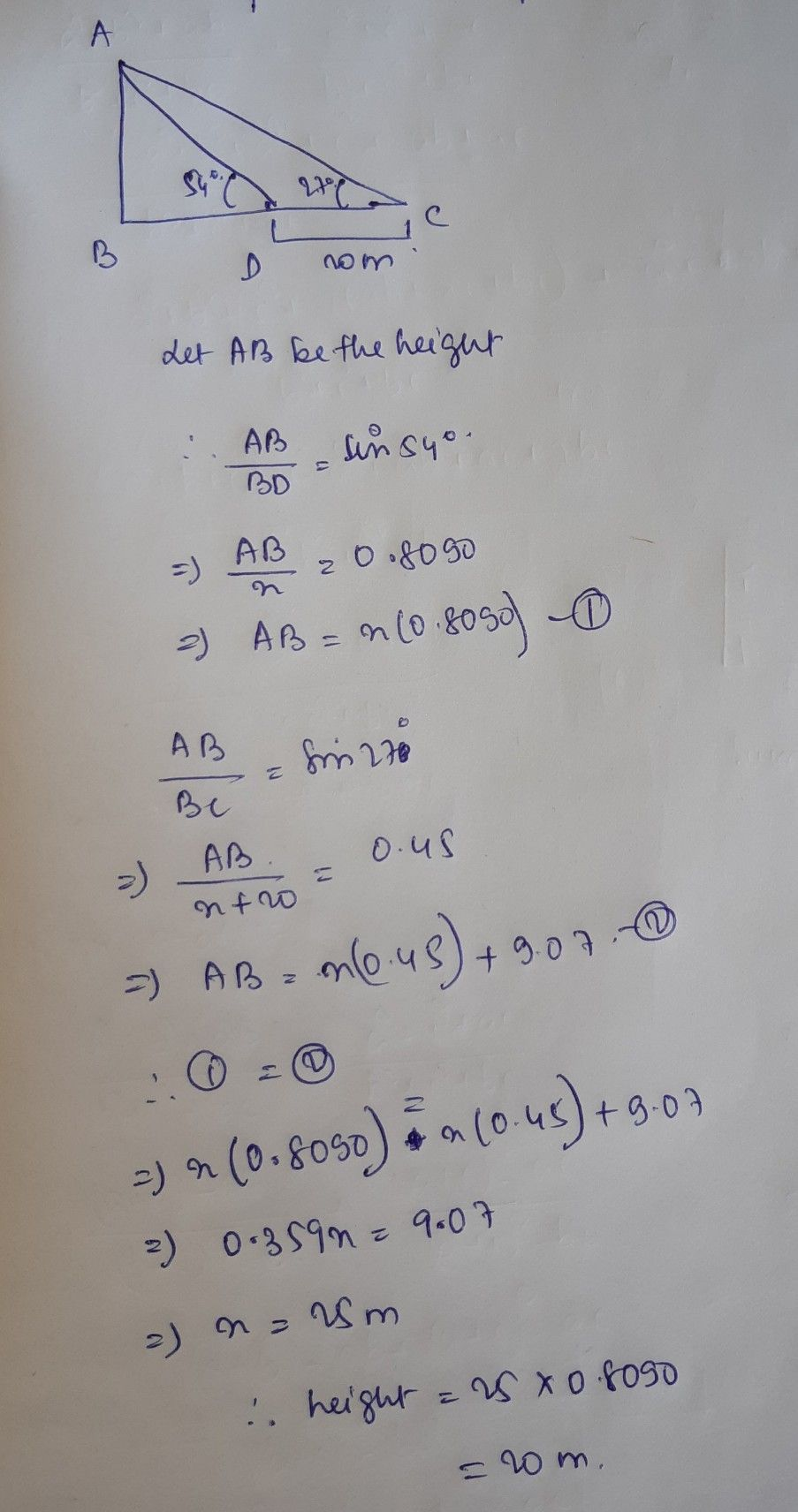Symbol
Problem$5$ A boy standing on the bank of a river sees the top of a tree on the other bank at an anele of elevation $540$ Stepping $20$ metres back, he sees it at an angle of elevation $270$ Find the height of the tree. $\left(sin27=0.45$ $cos27=0.89,tan27=0.51$ $sin54=0.80,cos54=0.59,tan54=1.38\right)$